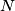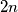### IMO Shortlist 2004 problem C5

Kvaliteta:
Avg: 0,0
Težina:
Avg: 8,0
3.$A$ and$B$ play a game, given an integer$N$,$A$ writes down$1$ first, then every player sees the last number written and if it is$n$ then in his turn he writes$n+1$ or$2n$, but his number cannot be bigger than$N$. The player who writes$N$ wins!, For wich values of$N$ does$B$ wins?
Izvor: Međunarodna matematička olimpijada, shortlist 2004